# MATLAB程序流程控制

• 顺序结构程序
• 用if语句实现选择结构
• 用switch语句实现选择结构
• 用for语句实现循环结构
• 用while语句实现循环结构
• 函数文件的定义与调用
• 函数的递归调用
• 函数参数与变量的作用域

1.分析问题，确定求解问题的数学模型或方法
2.设计算法，并画出流程图
3.选择编译工具，根据算法编写程序
4.调试程序，分析程序输出结果

• 顺序结构
• 选择结构
• 循环结构

• 脚本文件是可在命令行窗口执行的文件，也叫命令文件
• 函数文件是定义一个函数，不能直接执行，而必须以函数调用的方式来调用它

• 选择左上角的新建文本
• 用edit命令来创建文件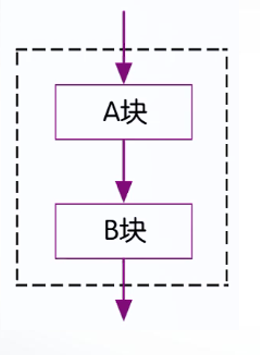• 数据的输入
A = input（提示信息，选项）
》A = input （‘请输入变量A的值:’）；
请输入变量A的值：100
• 数据的输出
disp(输出项)
》s = ‘Hello,World’;
》disp(s)
Hello ,World
• 程序的暂停
pause(延迟秒数)
若要强行终止程序的运行可使用Ctrl + C命令
• 输入原始数据
• 对原始数据进行处理
• 输出处理的结果

A（1，1） =》 1+i
B（4.5，4.5）=》 4.5+4.5i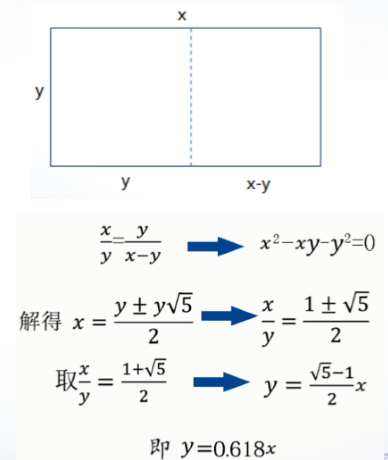a = input (‘a=’);
b = input (‘b=’);
c = a + 0.618*(b-a);
s = abs(a-b);
disp(s)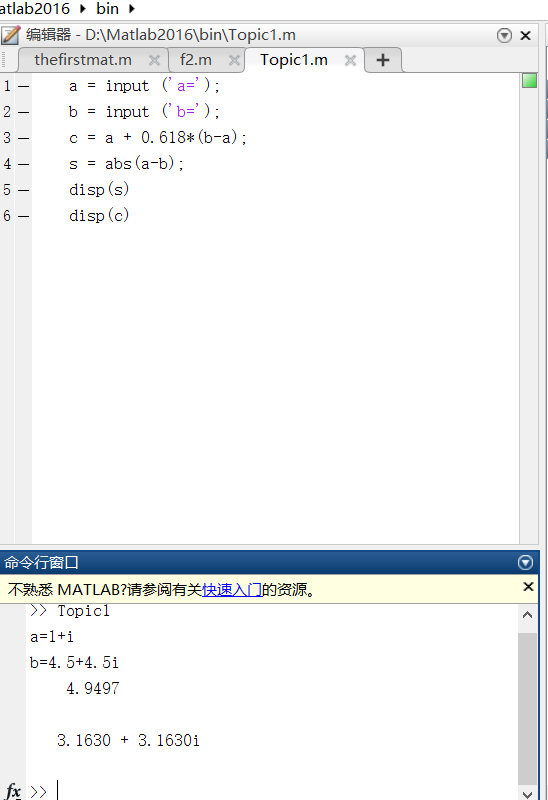• 什么是选择结构
• 单分支if语句
• 双分支if语句
• 多分枝if语句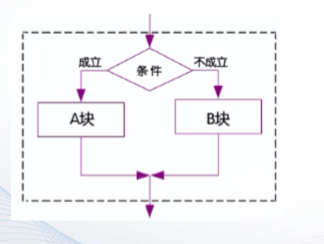• 用if语句实现选择结构
• 用switch语句实现选择结构

if 条件 关系运算或逻辑运算

end

• 当条件结果为标量时，非零表示条件成立，零表示条件不成立
• 当条件结果为矩阵时，如果矩阵为非空，且不包含零元素，则条件成立，否则不成立

[1,2;3,4]表示条件时，条件成立

if 条件

else

end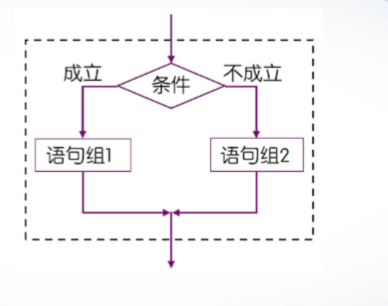x = input('请输入x的值:');
if  rem(x,2)==1
y = sqrt(x);
else
y=x^(1/3);
end
disp(y)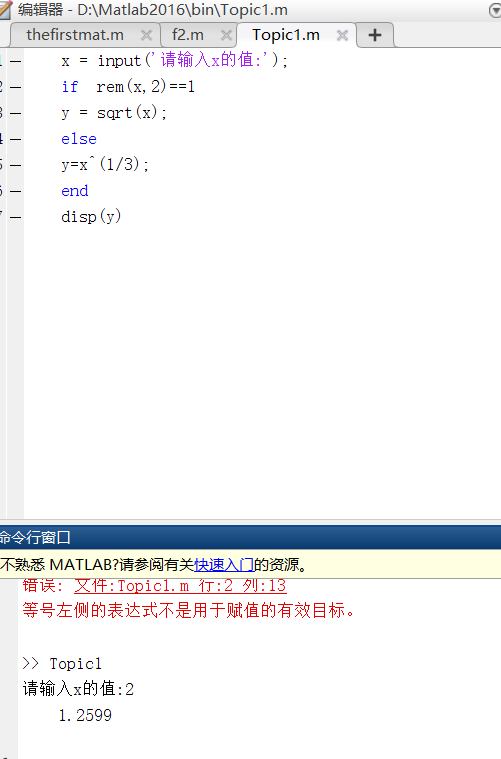if 条件1

else if 条件2

else if 条件m

else

end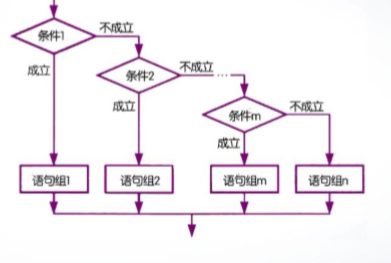c  = input('请输入一个字符: ','s');
if c >= 'A' && c <= 'Z'
disp(lower(c))
elseif c >= 'a'  && c <=  'z'
disp(upper(c))
elseif c >= '0'   && c <= '9'
disp(str2double(c)^2)
else
disp(c)
end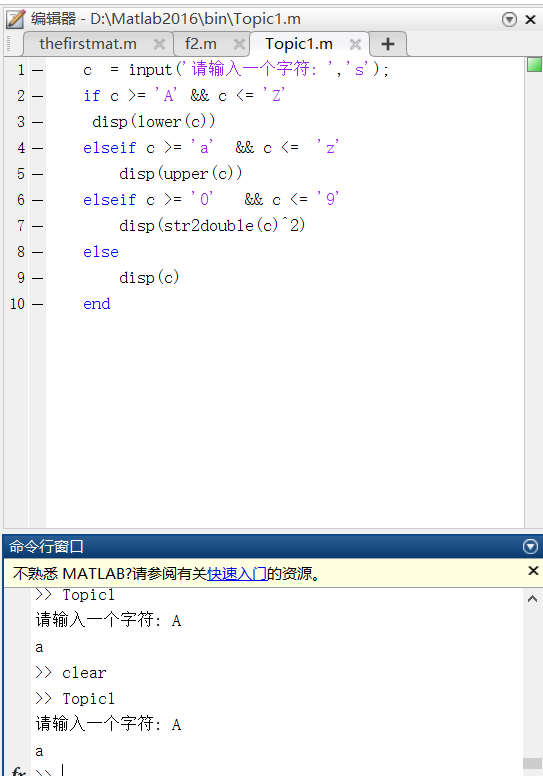• 语句格式
• 执行过程
• 实例

switch 表达式
case 结果表1

case 结果表2

case 结果表m

otherwise

end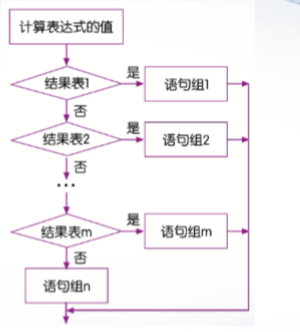• switch表达式应该时一个其值可以列举的表达式子
• case结果表为switch表达式的取值，当取值有多个时，用单元数据表示

c = input ('请输入一个单词：'，'s');
switch c (1)
case{'A','E','I','O','a','e','i','o','u'}
disp([c,'以元音字母开头']);
otherwise
disp([c,'以辅音字母开头']);
end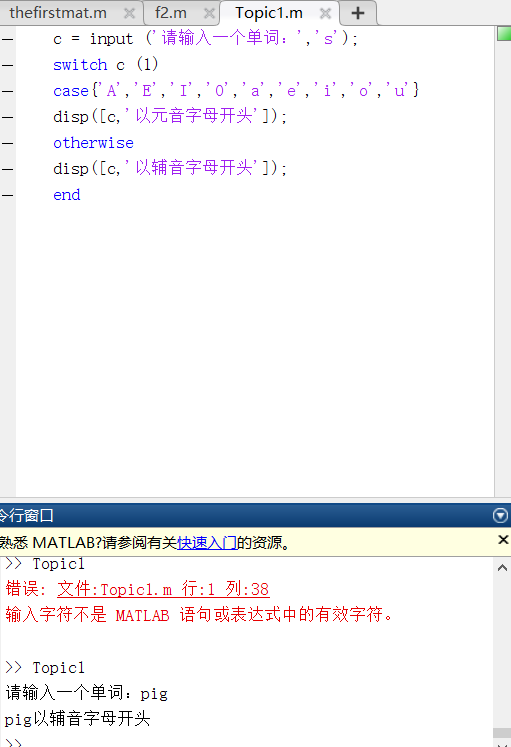• 什么是循环结构
• for语句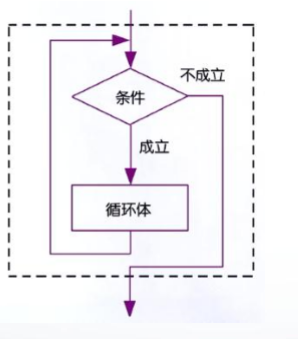for语句

for 循环变量=表达式1:表示2:表示3

end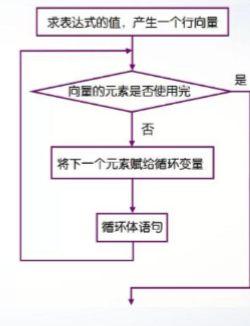• for语句针对向量的每一行元素执行一次循环体
for k[1,3,2,5]
k
end
• 退出循环之后，循环变量的值就是向量中最后的元素值
for k=1:2:10
end
k
• 当向量为空时，循环体一次也不执行
for k=1:-2:10
k
end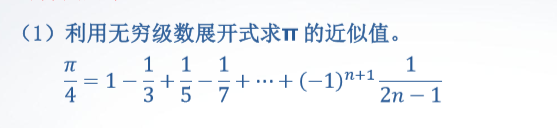y = 0;
g=-1;
n = input('n=?');
for i= 1:n
g = -g;
y = y + g/(2*i-1);
end
pai=4*y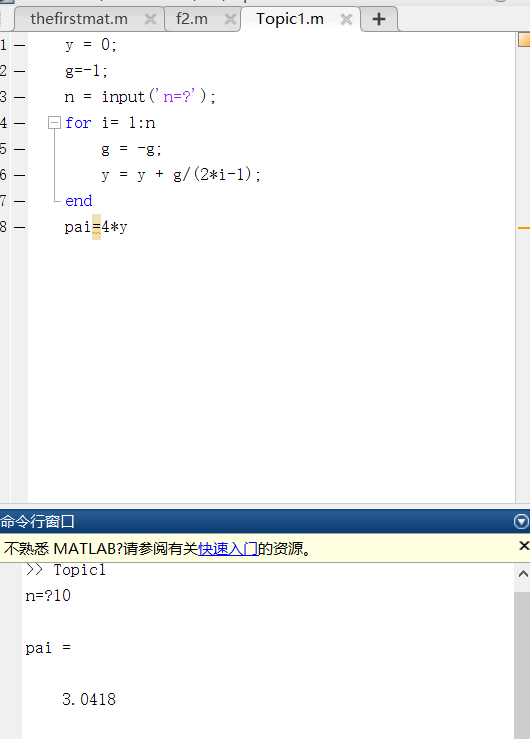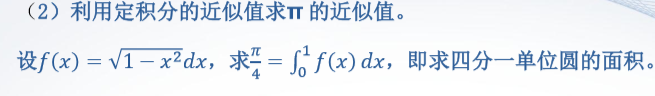a = 0;
b = 1;
n = input('n=?');
h = (b - a)/n;
x = a:h:b;
f = sqrt(1-x.*x);
s=[];
for k=1:n
s1=(f(k)+f(k+1))*h/2'
s=[s,s1];
end
pai=4*sum(s)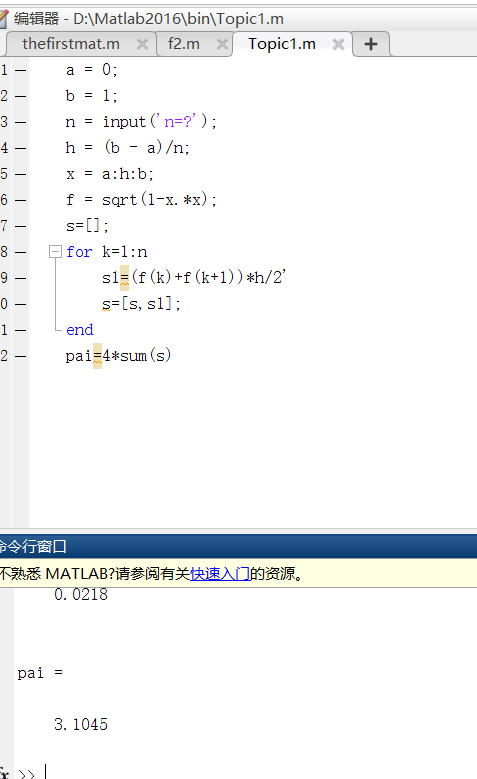for语句更一般的格式为：
for 循环变量=矩阵表达式

end

for k=[1,2,3,4]

for k=[1;2;3;4]

• while语句
• break语句和continue语句
• 循环的嵌套

while语句

end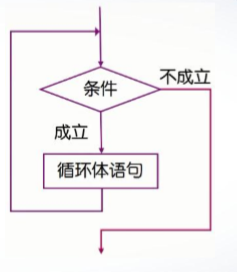msum = 0
n = 0
x = input('Enter a number (end in 0):');
while x~=0
msum = msum +x;
n = n+1;
x = input('Enter a number(end in 0):');
end
if n > 0
msum
mean=msum/n
end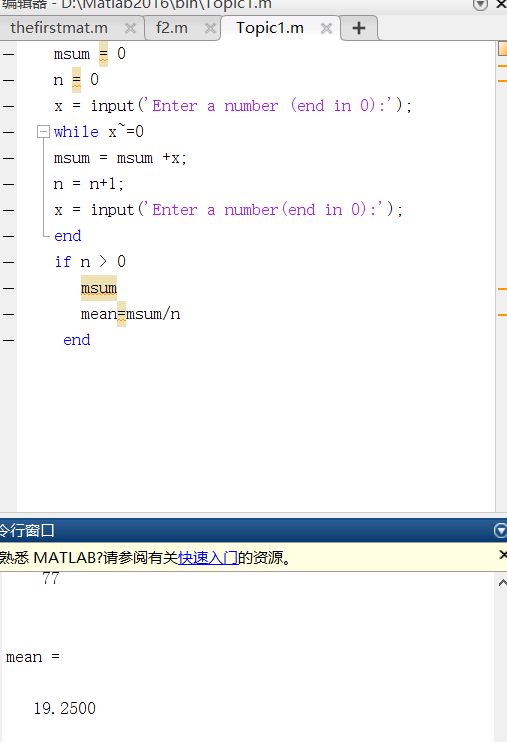• while语句多用于循环次数不确定的情况，而对于循环次数确定的情况，使用for语句更方便
• 针对不同情况可以选择不同的循环语句，但从功能上讲两种循环语句可以互相替代

break语句和continue语句

• break语句用来跳出循环体，结束整个循环体
• continue语句用来结束本次循环，接着进行下一次是否执行循环的判断

for n =100:200
if rem(n,21) ~=0
continue
end
n
break
end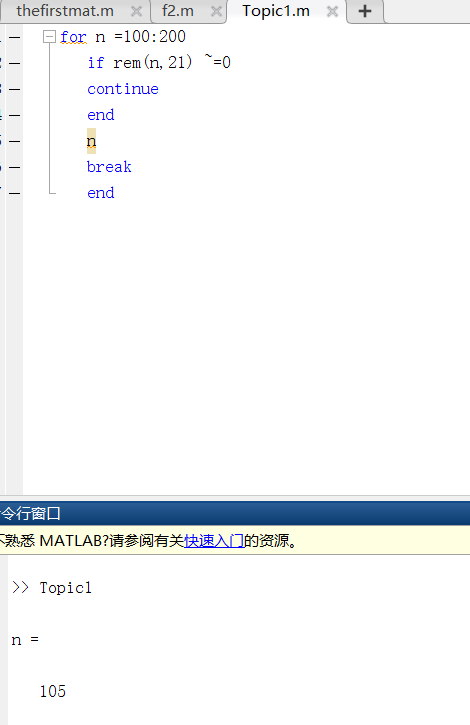m = input('m=');
p= 1:m;
p(1)=0;
for i =2:sqrt(m)
for j= 2*i:i:m
p(j)=0
end
end
n=find(p~=0);
p(n)


• 函数文件的基本结构
• 函数调用
• 匿名函数

function 输出形参表=函数名（输入形参表）

• 函数文件名通常由函数名再加上扩展名.m组成，函数文件名与函数名也可以不相同。当函数文件名与函数各不相同时，MATLAB会忽略函数名，调用使用函数文件名
• return语句表示结束函数的执行。通常，在函数文件中也可以不使用return语句，那么被调用函数执行完成后会自动返回

function [s,p] =fcircle(r)
s=pi*r*r;
p=2*pi*r;


[输出实参表]=函数名（输入实参表）

>>[s,p]=fcicle(10)


>>f=@(x,y) x^2+y^2
>>f(3,4)


>>h=@sin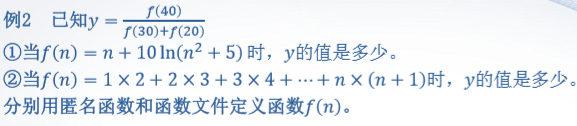f1=@(n)+10*log(n*n+5);
y1=f1(40)/(f1(30)+f2(20))
y2=f2(40)/(f2(30)+f2(20))

function f = f2(n)
f=0;
for k =1:n
f=f+k*(k+1);
end


• 函数的嵌套调用
• 函数的递归调用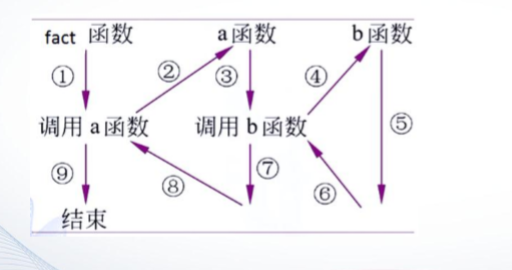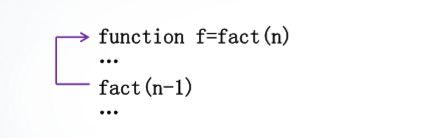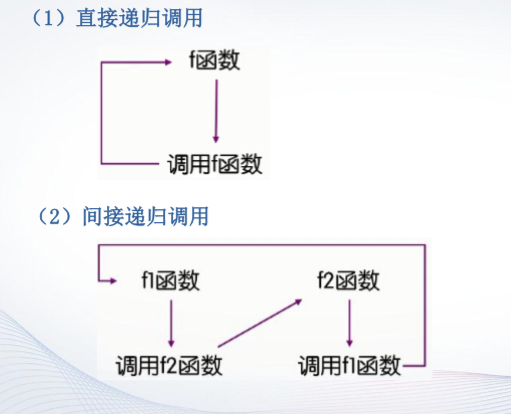• 函数参数的可调性
• 全局变量与局部变量

nargin ==> 输入实参的个数
nargout ==>输出实参的个数

• 全局变量：所有函数都可以对它进行存取和修改
• 局部变量：在程序中只在特定过程或函数中可以访问的变量

global 变量名©️2019 CSDN 皮肤主题: 精致技术 设计师: CSDN官方博客